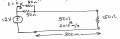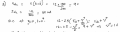# Transient Transmission Line Problem

#### betting5

Joined Dec 30, 2012
2
Hi all,

I need some help analyzing the circuit below. I understand that at t=0- the steady state Load Voltage is 9 V, and that when the switch is thrown it needs to bounce to 10.285 V, but I can't figure out how to get to the first voltage propagation (V+).My first try is to start at t=0+, R_eq = 25 Ohms, then V = 12 V * (Z0/(Z0+R_eq)) to get 8 V, but bouncing from this value puts me below 9V when i know that the value at infinity should be 10.285V.

My professor gave us solutions to the homework, but i don't know where he got the equation for V+. I've included his equation below:I don't need help with bouncing the voltage, just getting the conditions at t=0+ (i.e. i need to find V+ after 0). Any help would be appreciated.

-Barry

#### MrAl

Joined Jun 17, 2014
9,755
Hi,

A few questions...
So your transmission line is lossless?
Is that a 150 ohm load?
What position along the line are you solving for?

#### Tesla23

Joined May 10, 2009
524
Hi all,

I need some help analyzing the circuit below. I understand that at t=0- the steady state Load Voltage is 9 V, and that when the switch is thrown it needs to bounce to 10.285 V, but I can't figure out how to get to the first voltage propagation (V+).

View attachment 136221

My first try is to start at t=0+, R_eq = 25 Ohms, then V = 12 V * (Z0/(Z0+R_eq)) to get 8 V, but bouncing from this value puts me below 9V when i know that the value at infinity should be 10.285V.

My professor gave us solutions to the homework, but i don't know where he got the equation for V+. I've included his equation below:

View attachment 136222

I don't need help with bouncing the voltage, just getting the conditions at t=0+ (i.e. i need to find V+ after 0). Any help would be appreciated.